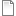# Comparison of two semantic approaches to unification

 Title Comparison of two semantic approaches to unification Publication Type Thesis Year of Publication 1996 Authors Leucker, M University RWTH Aachen Thesis Type masters Abstract The master theses compares the two most prominent semantic approaches to unifica tion. We can show that unification in primal algebras is not a direct instance of unification in monoidal theories. However, it is possible to reduce unification in a given primal algebra to unification in a corresponding monoidal theory. As by-products of this work we have shown that unification in algebras is an instance of unification modulo equational theories, and we have introduced a new notion of equivalence for equational theories.
Bibtex:
```@mastersthesis {Leucker96,
title = {Comparison of two semantic approaches to unification},
year = {1996},
school = {RWTH Aachen},
type = {masters},
abstract = {The master theses compares the two most prominent semantic approaches to unifica tion. We can show that unification in primal algebras is not a direct instance of unification in monoidal theories. However, it is possible to reduce unification in a given primal algebra to unification in a corresponding monoidal theory. As by-products of this work we have shown that unification in algebras is an instance of unification modulo equational theories, and we have introduced a new notion of equivalence for equational theories.},
author = {Martin Leucker}
}```
Postscript:diplom.ps_1.gz